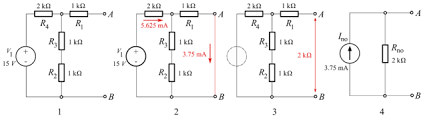Thevenin's Theorem and Norton's Theorem - Black keyhole

# Black keyhole

welcome to our keyhole, we provide you various knowledge from various sources such as Electric&Electronic, Health, Arduino, IOT, Diy projects, A/l past paper and model paper, Even though you can keep in touch with current technology

Thevenin's TheoremThevenin's theorem states it is possible to simplify any linear circuit. No matter how hard is it to an equivalent circuit with just a single voltage source with and resistor in series connected to a load. Thevenin's theorem is especially useful in analyzing power systems and other circuits where one particular resistor in the circuit is subject to change, and re-calculation of the circuit is necessary with each trial value of load resistance, to determine the voltage across it and current through it. and re-calculation of the circuit is necessary with each trail value of load resistance, to determine the voltage across it and current through it. This thevenient theorem provides easy way to find the voltage and current across the load without having to recalculate your entire circutit.

The two-terminal network containing Emf and R0 resistance in a series replaced as Thevenin's equivalent circuit.

To find the Thevenin Equivalent circuit follow the following process

1. Remove the Load between the terminal which you want to find the Thevenin equivalent circuit

2. Find the open-circuit voltage between the terminal. which is Thevenin voltage.

3. Determine the resistance between open circuit terminal with includes all ideal voltage sources and current. which is denoted by Ro. This is called Thevenin resistance

4. Connect the E0 and R0 in series to produce the Thevenin equivalent circuit across the two terminals.

5. Again connect the load removed in steps one and find the current across the load using ohm's law. That current will remain the same that what will be shown in original circuit.

Norton's theorem

In a close box which brought up with A and B terminal, which is contained with any number of network. It can contain any number of resistance, emf sources in any manner whether it is serial or parallel. but in Norton's theorem the circuit output with I_n_ current which has parallel resistance Rn. The Rn resistance is same Thevenin's Ro. once the norton equivellent circuit is determined , we can easily obtained the load RL value applied across the terminal  AB.Norton's theorem as applied to d.c circuit may be stated as follows

Any network having two terminals with A and B can be replaced with the output current source of I_n_ in parallel with the Resistance Rn

1.  The output I_n_ of the current source is equal to the current flow across the terminals AB when terminals A and B are short-circuited.

2. the resistance Rn is the resistance of a network between the terminal A and B with load removed Rl and sources of e.m.f replaced by their internal resistance.

if Norton's theorem is converse of Thevenin's theorem the Norton's theorem uses a current generator instead of  voltage generator and resistance Rn (which is same as Ro in Thevinin's) parallel with generator instead of series with the circuit.

Procedure for finding Norton Equivalent circuit

1. Open the terminal between which you want to find Norton's equivalent circuit.

2.  Short circuit the terminals which you opened under the consideration. find the out put current flowing through the short circuit terminals

3. find the resistance between the two opened terminals with includes all ideal voltage source shorted and all ideal current opened. (non ideal source is replaced by its internal resistance). This resistance is called Rn. It is easy to say that Rn=Ro

4. connect the  I_n_ current and Rn resistance in parallel to produce the Norton's equivalent circuit between the two terminals under consideration.